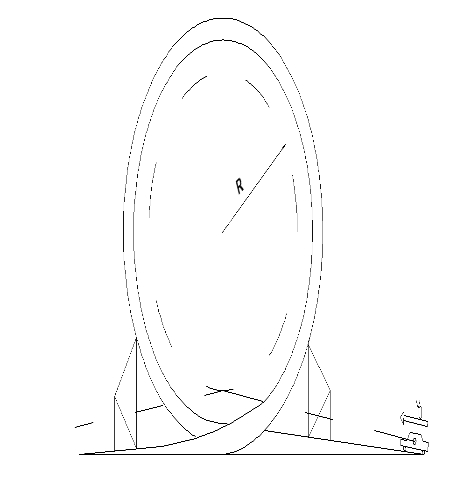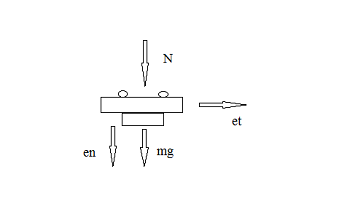# A stunt drivers wants to drive a car through the circular loop of radius R=5m. determine the...

## Question:

A stunt drivers wants to drive a car through the circular loop of radius R=5m. determine the minimum velocity at which the car can enter the loop and coast without losing contact with the track. What is the car?s velocity at the top of the loop?

Solve the problem to find the minimum initial velocity . Let?s define the angle that the car has travelled through the loop. ( =0 when the car enters the loop and = when car exits the loop.)

1) Plot the speed of the car as a function of

2) Use a free diagram (FBD) to find and plot the normal force as a function of .## Centripetal force:

A centripetal force makes a body to follow a curved path.Its direction is towards the instantaneous center of curvature of the path.A circular motion is an example where centripetal force acts.

Become a Study.com member to unlock this answer! Create your account{eq}V_{T}=V_{Top} \\\sum F_{n}=N+mg=\dfrac{mV_{T}^{2}}{R} {/eq}

For minimum velocity

{eq}mg=\dfrac{mV_{T}^{2}}{R} \\V...Centripetal Force: Definition, Formula & Examples

from

Chapter 8 / Lesson 2
252K

When an object is traveling in a circular path, centripetal force is what keeps it fixed in that path. Learn more about this force, how it is calculated and examples of its occurrence. A quiz is provided to test your learning.